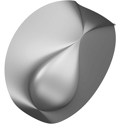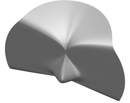Jean Constant

# Resource

### The following text & equations come directly from the Surfer program explanation and formula display. The software can be downloaded for free on the MFO-Imaginary site. The figures can be recreated exploring various x, y & z rotation effects.

Click the image on the right to go back to the gallery view.

### 01. Quaste – the ABC of an equation of Cartesian nature. Monomials of degree 1 are x, y, z.  Monomials of degree 2 are x2 , xy, y2, yz, z2, etc….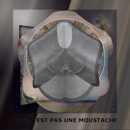### 02. Quaste #2### 03. Quaste #3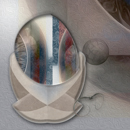### 04. Chmutov Octic. Equation written by SV Chmutov in the early 80s. At the time it constituted the world record for μ(d) most d. Beginning numbers have been replaced by some of the Fibonacci numbers sequence.### 05 Chmutov octic #2 on a vis a vis background disk. The cusp on the surface is a singularity. Black holes and the Big Bang constitute singularities cosmological model equations. The original equation has been altered with reverse Fibonacci numbers sequence.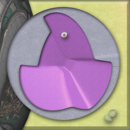### 06 Chmutov Octic #3 . Variation on the Chmutov octic equation that brings up its symmetrical aspect. Included, a parabola.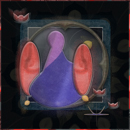### 07 RGB. Two spheres. Same object different color. Each as a distinct identity according to the RGB color model .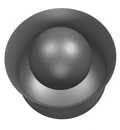### #1,#2, #3 Random numbers variation on the following equation